# Texas Go Math Grade 8 Lesson 10.1 Answer Key Surface Area of Prisms

Refer to our Texas Go Math Grade 8 Answer Key Pdf to score good marks in the exams. Test yourself by practicing the problems from Texas Go Math Grade 8 Lesson 10.1 Answer Key Surface Area of Prisms.

## Texas Go Math Grade 8 Lesson 10.1 Answer Key Surface Area of Prisms

Question 1.
Sara is lining the bottom and lateral faces of a drawer with liner paper. The dimensions of the inside of the drawer are 1 yard, 20 inches, and 9 inches. What is the total area in square inches being covered?
Given,
The dimensions of the inside of the drawer are 1 yard, 20 inches, and 9 inches.
1 yard = 36 inches
We know that,
Total surface area of the prism = 2B + PH
TSA = 2 (36 × 12) + 112 (1)
TSA = 2(720) + 112
TSA = 1552 sq. in
Thus the total surface area is 1552 sq. in

Find the lateral area and total surface area of each prism.

Question 2.Height = 10 cm
Lateral Surface Area of the prism = Perimeter × height
Perimeter = 8 + 6 + 10 = 24 cm
LSA = 24 × 10 = 240 sq. cm
Total surface area of the prism = 2B + PH
B = area of base
A = 1/2 × b × h
A = 1/2 × 8 × 6
A = 24 sq. cm
TSA = 2 (24) + 24 (10)
TSA = 48 + 240
TSA = 288 sq. cm
Thus the Lateral Surface Area of the prism is 240 sq. cm and the Total surface area of the prism is 288 sq. cm

Question 3.Given,
height = 6 in.
Lateral Surface Area of the prism = Perimeter × height
Perimeter = 5 + 6.5 + 6 = 17.5
LSA = 17.5 × 6 = 105 sq. in.
Total surface area of the prism = 2B + PH
B = area of base
A = 1/2 × b × h
A = 1/2 × 5 × 6
A = 15
Total surface area of the prism = 2B + PH
TSA = 2 × 15 + 17.5 × 6
TSA = 30 + 105
TSA = 135 sq. in
Thus the Lateral Surface Area of the prism is 105 sq. in. and the Total surface area of the prism is 135 sq. in

Find the lateral area and total surface area of each prism. (Example 1)

Question 1.Given,
length = 5 in.
width = 4 in.
height = 9 in.
Lateral Surface Area of the prism = Perimeter × height
Perimeter of the base = 2l + 2w
P = 2 × 5 + 2 × 4
P = 10 + 8
P = 18 in
LSA = PH
LSA = 18 × 9
LSA = 162 sq. in
Total surface area of the prism = 2B + PH
B = area of base
Area of base = l . w
A = 5 × 4
A = 20 sq. in
Total surface area of the prism = 2B + PH
TSA = 2 × 20 + 162
TSA = 40 + 162
TSA = 202 sq. in
Thus the Lateral Surface Area of the prism is 162 sq. in. and the Total surface area of the prism is 202 sq. in

Question 2.Given,
length = 2 yd or 6 ft
width = 4 ft.
height = 5 ft.
Lateral Surface Area of the prism = Perimeter × height
Perimeter of the base = 2l + 2w
P = 2 × 6 + 2 × 4
P = 12 + 8
P = 20 ft.
LSA = 20 × 5
LSA = 100 sq. ft
Total surface area of the prism = 2B + PH
B = area of base
Area of base = l . w
A = 6 × 4
A = 24 sq. ft
Total surface area of the prism = 2B + PH
TSA = 2 × 24 + 100
TSA = 48 + 100
TSA = 148 sq. ft
Thus the Lateral Surface Area of the prism is 100 sq. ft. and the Total surface area of the prism is 148 sq. ft

Question 3.
Akira plans to cover the box shown in contact paper. Find the amount of contact paper that Akira needs, not counting overlap. (Example 1)Given,
length = 9 in.
width = 7 in.
height = 4 in.
We know that,
Lateral Surface Area of the prism = Perimeter × height
Perimeter of the base = 2l + 2w
P = 2 × 9 + 2 × 7
P = 18 + 14
P = 32 in.
LSA = 32 × 4
LSA = 128 sq. in.
Total surface area of the prism = 2B + PH
B = area of base
Area of base = l . w
A = 9 × 7
A = 63 sq. in.
Total surface area of the prism = 2B + PH
TSA = 2 × 63 + 128
TSA = 126 + 128
TSA = 254 sq. in
Thus the Lateral Surface Area of the prism is 128 sq. in. and the Total surface area of the prism is 254 sq. in.

Question 4.
A gift box is in the shape of a triangular prism. How much cardboard is needed to construct the box, not counting overlap? (Example 2)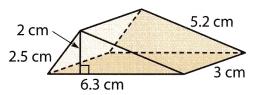Area of the base = 1/2 × b × h
A = 1/2 × 6.3 × 2
A = 6.3 sq. cm
The perimeter of the base = 6.3 + 5.2 + 2.5 = 14 cm
Lateral Surface Area of the prism = Perimeter × height
LSA = 14 × 2
LSA = 28 sq. cm
Total surface area of the prism = 2B + PH
TSA = 2 × 6.3 + 28
TSA = 12.6 + 28
TSA = 40.6 sq. cm
Thus the Lateral Surface Area of the prism is 28 sq. cm. and the Total surface area of the prism is 40.6 sq. cm.

Essential Question Check-In

Question 5.
What are two ways that you can find the surface area of a prism?
The surface area can be calculated in one of two ways.
One way involves using an equation for the lateral area.
Another way involves taking the area of all the sides and summing the areas.

Find the lateral and total surface area of each prism. Round to the nearest tenth if necessary.

Question 6.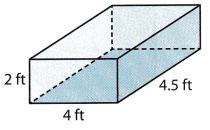Given,
length = 4 ft.
width = 4.5 ft.
height = 2 ft.
We know that,
Lateral Surface Area of the prism = Perimeter × height
Perimeter of the base = 2l + 2w
P = 2 × 4 + 2 × 4.5
P = 8 + 9
P = 17 ft.
Lateral Surface Area of the prism = Perimeter × height
LSA = 17 × 2
LSA = 34 sq. ft
Total surface area of the prism = 2B + PH
B = area of base
Area of base = l . w
A = 4 × 4.5
A = 18 sq. ft.
Total surface area of the prism = 2B + PH
TSA = 2 × 18 + 34
TSA = 36 + 34
TSA = 70 sq. ft
Thus the Lateral Surface Area of the prism is 34 sq. ft. and the Total surface area of the prism is 70 sq. ft.

Question 7.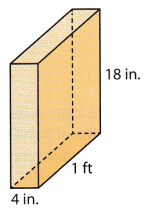Given,
length = 4 in.
width = 1 ft = 12 in.
height = 18 in.
We know that,
Lateral Surface Area of the prism = Perimeter × height
Perimeter of the base = 2l + 2w
P = 2 × 4 + 2 × 12
P = 8 + 24
P = 32 in.
Lateral Surface Area of the prism = Perimeter × height
LSA = 32 × 18
LSA = 576 sq. in
Total surface area of the prism = 2B + PH
B = area of base
Area of base = l . w
A = 4 × 12
A = 48 sq. in.
Total surface area of the prism = 2B + PH
TSA = 2 × 48 + 576
TSA = 96 + 576
TSA = 672 sq. in.
Thus the Lateral Surface Area of the prism is 576 sq. in. and the Total surface area of the prism is 672 sq. in.

Question 8.Given,
length = 6 cm
width = 80 mm = 8 cm
height = 9 cm
We know that,
Lateral Surface Area of the prism = Perimeter × height
Perimeter of the base = 2l + 2w
P = 2 × 6 + 2 × 8
P = 12 + 16
P = 28 cm
Lateral Surface Area of the prism = Perimeter × height
LSA = 28 × 9
LSA = 252 sq. cm
Total surface area of the prism = 2B + PH
B = area of base
Area of base = l . w
A = 6 × 8
A = 48 sq. cm.
Total surface area of the prism = 2B + PH
TSA = 2 × 48 + 252
TSA = 96 + 252
TSA = 348 sq. cm.
Thus the Lateral Surface Area of the prism is 252 sq. cm. and the Total surface area of the prism is 348 sq. cm.

Question 9.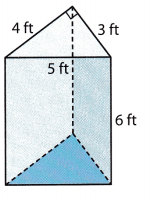The surface area of the triangular prism = bh + (b1 + b2 + b3)l
h = 4 ft
b =5 ft
b1 = 5 ft
b2 = 4 ft
b3 = 3 ft
l = 6 ft
= 5(4) + (5 + 4 + 3)6
= 20 + 72
= 92 sq.ft.
The surface area of the triangular prism = 92 sq.ft.
Lateral surface area of the triangular prism = (a + b + c)h
a = 5 ft
b = 4 ft
c = 3 ft
h = 6 ft
= (5 + 4 + 3)6 = 72 sq. meter.
Lateral surface area of the triangular prism = 72 sq.meter.

Question 10.The shape is in the form of triangular prism
First find the area of the triangle for 1 base = ½ x bh
Breath = b = 8 cm
Height = h = 7.5 cm
Area of the rectangle for 1 base = ½ × 8 × 7.5 = 30 sq. meter.
Area of the rectangle for 2 bases = 30 × 2 = 60 sq. meter.
Second, find the area of the rectangle for 1 side = b × h
Breath = b = 5 cm.
Height = h = 8.5 cm.
= 5 × 8.5 = 42.5 sq. meter.
For second side = 2 × 42.5 = 85 sq.meter.
For bottom = b × h = 8 × 8.5 = 68 sq.meter.
The total surface area = 60 + 85 + 68 = 213 sq. meter
Lateral surface area of the triangular prism = (a + b + c)h
a = 8 cm
b = 8.5 cm
c = 5 cm
h = 7.5
= (8 + 8.5 + 5)7.5 = 161.25 sq. meter.

Question 11.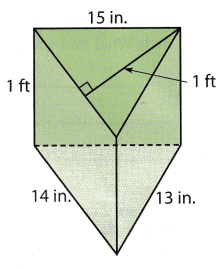Formula for the surface area of a triangle = SA = ph + 2B
P = 15 + 14 + 13 = 42
H = 1 ft = 12 inches
B = ½ bh
B = ½ x 42 x 12 = 252 sq. inches.
SA = ph + 2B
SA = 42 x 12 + 2(252)
SA for triangle = 1008 sq. inches.
Surface area of the rectangle
SA = 2(h + b) = 2(12 + 14) = 52 sq. inches.
Surface area of the triangular prism = surface area of a triangle + surface area of the rectangle
= 1008 + 52 = 1060 square inches.
Lateral surface area of the triangular prism = (a + b + c)h.
a = 15 inches.
b = 14 inches.
c = 13 inches.
h = 12 inches.
= (15 + 14 + 13)12 = 504 sq. meter.

Question 12.
The bases of a prism are right triangles with side lengths 6 meters, 8 meters, and 10 meters. The height of the prism is 3 meters. What is the lateral area of the prism? What is the total surface area?
Given,
The bases of a prism are right triangles with side lengths of 6 meters, 8 meters, and 10 meters.
The height of the prism is 3 meters.
S1 = 6 m
S2 = 8 m
S3 = 10 m
h = 3 m
Lateral Surface Area of the prism = Perimeter × height
Perimeter = 6 + 8 + 10 = 24 m
LSA = 3 × 24
LSA = 72 sq. meters
Total surface area of the prism = 2B + PH
B = area of base
B = (6m × 8m)/2 = 48/2 = 24 m²
SA = 2(24m²) + 72m²
SA = 48 m² + 72 m²
SA = 120 m²

Question 13.
A rectangular prism has a length of 8 inches and a width of 7 inches. The lateral area is 150 square inches. What is the height of the prism?
Given,
A rectangular prism has a length of 8 inches and a width of 7 inches.
The lateral area is 150 square inches
Lateral Surface Area of the prism = Perimeter × height
Perimeter of the base = 2l + 2w
P = 2 (7 + 8)
P = 2 × 15
P = 30
Lateral Surface Area of the prism = Perimeter × height
150 = 30 × h
h = 150/30
h = 5
Thus the height of the prism is 5 in.

Question 14.
Multiple Representations Write a formula for the total surface area of a cube in terms of its edge length x. Explain your reasoning.
the surface area of a cube
cube=6 squares
square=2 equal sides
area of square=side²
the surface area of cube=6(area of square)=6side²
side=x
SA=6x²

Question 15.
Multistep Malt bought a tent without a floor. Estimate the surface area of the tent in square feet.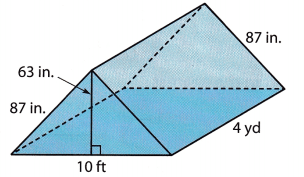The tent is in the shape of triangular prism
First find the area of the triangle for 1 base = ½ x bh
Breath = b = 10ft = 120 inches
Height = h = 63 inches
Area of the rectangle for 1 base = ½ × 120 × 63 = 3780 sq. inches.
Area of the rectangle for 2 bases = 3780 × 2 = 7560 sq. inches
Second find the area of the rectangle for 1 side = b × h
Breath = b = 87 inches
Height = h = 4 yard = 144 inches
= 87 × 144 = 12528 sq. inches.
For second side = 2 × 12528 = 25056 sq. inches.
For bottom = b × h = 120 × 63 = 7560 sq.inches.
The total surface area = 3780 + 25056 + 7560 = 36396 sq. inches
36396 sq. inches = 252.75 sq. feet.
The total surface area of the tent = 252.75 sq. feet.

Question 16.
Multistep Keeshawn is building a box with a lid out of plywood with the dimensions shown. Plywood costs $0.50 per square foot. Find the cost of the plywood Keeshawn needs for the box.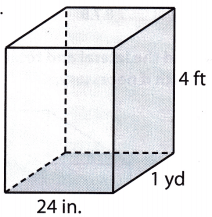Answer: Area of a box = l × b × h Length = 24 inches = 2ft Breadth = 1 yd = 3ft Height = 4ft = 2 × 3 × 4 = Area of the box = 24 square ft The cost of plywood =$0.50 per square foot
= 24 × $0.50 =$12.
The cost of plywood for the total box = \$12.

Question 17.
A glass prism on a chandelier is 75 millimeters long. The base of the prism is an equilateral triangle with side lengths of 9 millimeters and a height of about 7.8 millimeters. What is the approximate surface area of the prism?
Given,
A glass prism on a chandelier is 75 millimeters long.
The base of the prism is an equilateral triangle with side lengths of 9 millimeters and a height of about 7.8 millimeters
Area of the equilateral triangle:
1/2 × 7.8 × 9
There are two triangles – one on each end. So we multiply our previous expression by 2.
1/2 × 7.8 × 9 × 2
= 7.8 × 9
Each rectangle is 75 millimeters long and 9 millimeters wide: 75×9 sq. mm
We have 3 rectangles each the same size so we multiply 75×9 by 3.
75×9×3 = 2025
2025 + 70.2 = 2095.2 sq. mm

H.O.T. Focus on Higher Order Thinking

Question 18.
Problem Solving A cube with an edge length of 4 inches is painted on all of its sides. Then the cube is cut into 64 cubes with an edge length of 1 inch. What percent of the total surface area is painted? Explain.
Given,
A cube with an edge length of 4 inches is painted on all of its sides.
Then the cube is cut into 64 cubes with an edge length of 1 inch.
Find the surface area of the painted cube.
6(42) = 96 in².
Find the surface area of each of the smaller cubes.
6(12) = 6 in².
Find the total surface area of the smaller cubes.
64(6) = 384 in².
Find the percent of the total surface area painted.
96
384
= 0.25, or 25%.
The total surface area painted is 25%.

Question 19.
Problem Solving The base of a triangular prism is a right triangle whose legs are 7 cm and 24 cm. The height of the prism is 30 cm. What is the lateral area of the prism? Explain how you found your answer.
Given,
The base of a triangular prism is a right triangle whose legs are 7 cm and 24 cm.
The height of the prism is 30 cm.
The formula of a lateral area of a triangular prism is 1/2 × PH
The perimeter of a triangle is the total of all sides.
base² = 7² + 24²
base² = 625
b = 25 cm
Therefore base = 25 cm
Perimeter = 7 + 24 + 25 = 56 cm
LSA = 1/2 × 56 × 30 = 840 cm²
Therefore the lateral area of the prism is 840 cm²

Question 20.
Communicate Mathematical Ideas Explain how to find the surface area of the composite figure. Then find its surface area.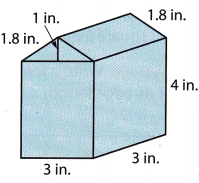Formula for the surface area for triangle = SA = ph + 2B
P = 1.8 + 1.8 + 3 = 6.6
H = 3 inches
B = ½ bh
B = ½ x 1 x 3 = 1.5
SA = ph + 2B
SA = 6.6 x 3 + 2(1.5)
SA for triangle = 22.8 sq. inches.
For square
P = 3 + 3 + 3 + 3 = 12
H = 4
B = 3 x 4 = 12
Square = SA = 12(4) + 2(12)
SA = 48 + 24
SA = 72 sq. inches.
Surface area of composite figure = surface area of a triangle + surface area of square
= 22.8 + 72 = 94.8 square inches.

Scroll to Top